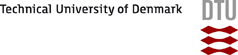DTU Risø Campus## How to formulate equilibria in Chemsimul

In CHEMSIMUL an equilibrium is determined by the rate constants of the forward and backward reactions and not solely by the equilibrium constant.

It is sometimes possible to describe acid/base equilibrium with different sets of chemical reactions.

For example

H2O2 = H[+] + H2O[-] ; k1

H[+] + H2O[-] = H2O2; k2

And

H2O2 + OH[-] = H2O[-] + H2O;k3

H2O[-] + H2O = H2O2 + OH[-];k4

You can use either set of reactions, but do not use both simultaneously.